# The diagonal of circle problems

#### Number of problems found: 34

• Company logoThe company logo consists of a blue circle with a radius of 4 cm, which is an inscribed white square. What is the area of the blue part of the logo?
• Axial sectionAxial section of the cylinder has a diagonal 40 cm. The size of the shell and the base surface are in the ratio 3:2. Calculate the volume and surface area of this cylinder.
• Axial sectionThe axial section of the cylinder has a diagonal 31 cm long, and we know that the area of the side and the base area is in ratio 3:2. Calculate the height and radius of the cylinder base.
• Hexagon rotationA regular hexagon of side 6 cm is rotated through 60° along a line passing through its longest diagonal. What is the volume of the figure thus generated?
• RectangleThe rectangle is 21 cm long and 38 cm wide. Determine the radius of the circle circumscribing rectangle.
• Logs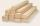The log has diameter 30 cm. What's largest beam with a rectangular cross-section can carve from it?
• Annular areaThe square with side a = 1 is inscribed and circumscribed by circles. Find the annular area.
• WasteHow many percents are waste from a circular plate with a radius of 1 m from which we cut a square with the highest area?
• Again saw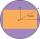From the trunk of the tree we have to a sculpture beam with rectangular cross-section with dimensions 146 mm and 128 mm. What is the trunk smallest diameter?
• Tree trunk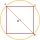What is the smallest diameter of a tree trunk that we can cut a square-section square with a side length of 20 cm?
• Round table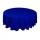Round table with diameter d = 105 cm is coated by square tablecloth with a side length 121 cm. About how many cm is higher center of tablecloth than its cornes?
• Draw a trapezoidDraw a trapezoid if given a = 7 cm, b = 4 cm, c = 3.5 cm, diagonal AC = 5cm. Solve as a construction task.
• Metal washersMetal washers with a diameter of 80 mm are cut from a strip of steel sheet with a width of 10 cm and a length of 2 m. Calculate the percentage of material waste if no material is lost when two adjacent circles meet.
• Mrak - cloud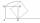It is given segment AB of length 12 cm, where one side of the square MRAK laid on it. MRAK's side length 2 cm shown. MRAK gradually flips along the line segment AB the point R leaves a paper trail. Draw the whole track of point R until square can do the l

We apologize, but in this category are not a lot of examples.
Do you have an interesting mathematical word problem that you can't solve it? Submit a math problem, and we can try to solve it.

We will send a solution to your e-mail address. Solved examples are also published here. Please enter the e-mail correctly and check whether you don't have a full mailbox.

Please do not submit problems from current active competitions such as Mathematical Olympiad, correspondence seminars etc...

Diagonal - math problems. Circle Problems.